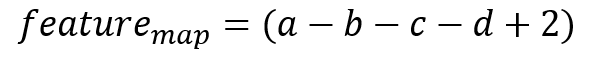# Question regarding CNN feature visualization

Hi there,

This my first post and I come with a question that might seem dumb to you but there is something I really don’t understand regarding CNN feature visualization.

You can often see (for example here https://github.com/utkuozbulak/pytorch-cnn-visualizations) codes to visualize CNN features that are based on the principle of “input optimization”:

1. You load a pre-trained model and freeze all its weights.
2. You create a random image.
3. You feed it into the network and compute a loss that is actually minus the mean value of the feature map corresponding to the filter you want to visualize. So the more the filter activation is high, the smaller is the loss.
4. You update the image thanks to this loss.

Let’s take a simple example. Here are the filter we are interested in and a random image.If we compute the feature map, we get a single element feature map:We see that to make the mean of the feature map the higher possible, we just have to choose the good signs for a, b, c and d and then go to infinity.

And this is actually the case if you choose a bigger image, you will just have to find the right signs and the input image will never converge.

I know there is something wrong in my reasoning as I guess this way of doing works, can you tell where is the issue ?

Thanks !
Rex

I think the error is here. As the filter slides over the image, ‘a’ will be multiplied by different elements of the filter, not always by 1.

A less important issue is that image pixels are in a bounded range, therefore the mean of activations converges to a maximum, not to infinity.

IMHO.

Hi Pomo, thanks for the answer.

My example was not complexe enough to show my problem. Let’s consider an image where the filter will slide on the image as you say.With this image, we will get the following feature map and loss:Once again, you just have to choose the right signs and I don’t see what could prevent the image pixels from going beyond their bounded range.

EDIT: actually, I would have thought about normalization but I don’t see any mention to normalization here for example: https://towardsdatascience.com/how-to-visualize-convolutional-features-in-40-lines-of-code-70b7d87b0030

Your argument looks good to me, but I’ve been wrong a couple of times before.

Your logic says that the mean activation of a convolution is a linear combination of the input pixel values. Therefore, the maximum activation will be found by pushing pixels to their extremes, according to their coefficients.

If you will take a suggestion, this conclusion is easy to verify numerically. Jeremy made an Excel spreadsheet that calculates a convolution. Try pushing a few pixels to their limits to see what happens to the filter mean. You may discover an error in your proof. Or your idea may be an actual truth about convolutions, certainly one I have never thought of and one that is very interesting. Thanks!

If it is true, the problem in your reasoning about CNN visualization must lie “upstream”. Of course, once the activations pass through a non-linear activation function or a pooling layer, the mean activation is no longer a simple function to calculate and maximize. Perhaps this is the issue - in a CNN, deeper convolutional outputs have already passed through many non-linearities.

Please let me know what you figure out!

For a filter in the first level (operating directly on the input pixels) this is right.
For a fixed set of convolution coefficients, the feature-map-mean is a linear function of the input pixels. As such, every input pixel has exactly one coefficient. If that coefficient is not zero, you can push the map-mean to infinity by pushing the input pixel to infinity (with regard to the sign of the coefficient).

However, I don’t see why this is a problem. We’re not looking for convergence of the optimization problem. If you push each pixel in the right direction (negative or positive infinity) then you’ll start seeing the desired picture. Pushing the pixels further towards the optimal solution (by running more iterations of the optimizer) won’t change that picture.

In the article you linked, the optimization is aborted after a fixed number of iterations, so that’s why the problem probably doesn’t occur. If you plan to do this for longer then yes, some sort of clipping/normalization will be necessary.